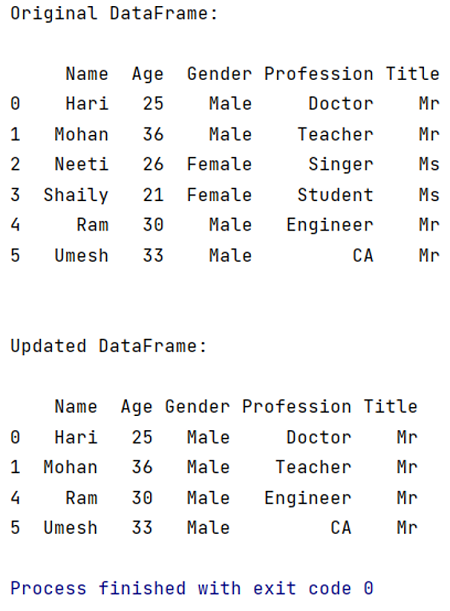# Deleting DataFrame row in Pandas based on column value

Given a panda DataFrame, we have to delete a row based on a column value.
Submitted by Pranit Sharma, on April 17, 2022

DataFrame rows are based on the index values. We can manipulate both rows and columns in pandas, DataFrame.drop() method is used to delete any particular row or column based on the condition which is passed as a parameter. Here, we are going to use DataFrame.drop() to delete a row based on a column value condition.

## pandas.DataFrame.drop() Method

This method is used to remove a specified row or column from the pandas DataFrame. Since rows and columns are based on index and axis values respectively, by passing the index or axis value inside DataFrame.drop() method we can delete that particular row or column. Below is the syntax:

Syntax:

```DataFrame.drop(
labels=None,
axis=0,
index=None,
columns=None,
level=None,
inplace=False,
errors='raise'
)

# short forms
df.drop(axis=None)  # deletes a specified column
df.drop(index=None) # deletes a specified row
```

To work with Python Pandas, we need to import the pandas library. Below is the syntax,

```import pandas as pd
```

Let us understand with the help of an example.

Example:

```# Importing pandas package
import pandas as pd

# Creating a dictionary
d = {
"Name":['Hari','Mohan','Neeti','Shaily','Ram','Umesh'],
"Age":[25,36,26,21,30,33],
"Gender":['Male','Male','Female','Female','Male','Male'],
"Profession":['Doctor','Teacher','Singer','Student','Engineer','CA'],
"Title":['Mr','Mr','Ms','Ms','Mr','Mr']
}

# Now, Create DataFrame
df = pd.DataFrame(d)

# Printing the original DataFrame
print("Original DataFrame:\n")
print(df,"\n\n")

# Now, Deletes a row where column Title is 'Ms'
# For this we will find the index value i.e.
# the row number where the column value is 'Ms'
index_to_be_deleted = df[ df['Title'] == 'Ms' ].index

# Now, Pass the index_to_be_deleted as a parameter inside
# df.drop() method to delete those rows having
# column value = 'Ms'
df.drop(index_to_be_deleted,inplace=True)

# Now, Printing the modified DataFrame
print("Updated DataFrame:\n")
print(df)
```

Output:

```Original DataFrame:

Name  Age  Gender Profession Title
0    Hari   25    Male     Doctor    Mr
1   Mohan   36    Male    Teacher    Mr
2   Neeti   26  Female     Singer    Ms
3  Shaily   21  Female    Student    Ms
4     Ram   30    Male   Engineer    Mr
5   Umesh   33    Male         CA    Mr

Updated DataFrame:

Name  Age Gender Profession Title
0   Hari   25   Male     Doctor    Mr
1  Mohan   36   Male    Teacher    Mr
4    Ram   30   Male   Engineer    Mr
5  Umesh   33   Male         CA    Mr
```Preparation